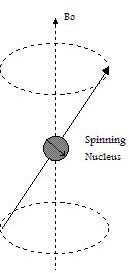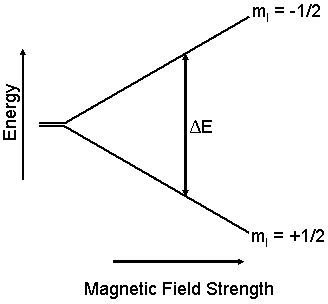# The Physics of Magnetic Resonance Imaging (MRI)

Since it is able to provide high contrast between soft tissues, Magnetic Resonance Imaging (MRI) has brought many improvements to diagnostic imaging in medical science. MRI technology is based on nuclear magnetic resonance spectroscopy (NMR), which is founded on the interaction between atomic nuclei with a nonzero magnetic moment and an external magnetic field. In order to understand how MRI performs, one should understand the phenomenon of NMR.

## Nuclear Magnetic Resonance (NMR)

Subatomic particles such as protons, neutrons, and electrons have a fundamental characteristic property called spin, which can be regarded as an angular momentum. According to quantum mechanics, the spin contains information about direction and the component of spin along a certain direction can take only discrete values. Protons and neutrons are fermions, particles which have half-integer spin and which obey the Pauli exclusion principle.

In some atoms the nuclear spins are paired and that results in no overall spin for the nucleus. However, if the nucleus contains an odd number of protons and/or neutrons, it has an intrinsic angular momentum and magnetic moment. If a nucleus with unpaired spins is placed in a magnetic field, the nuclear magnetic moment and the external field will interact (see figure at left). This interaction is explained by the classical theory of electromagnetism as a precession of such nuclear moments about the (static) magnetic field Bo at an angular frequency ωo (Larmor frequency).The nuclear magnetic moment tends to align with the applied field in a parallel (lower energy) or anti-parallel (higher energy) orientation, but precess about this field at a certain angle, with angular frequency given by the following equation:

ωo = γ.Bo

where γ is the gyromagnetic ratio, a constant number for a particular type of nucleus.

Once there is an energy difference between two spin states, it is possible to induce transitions from one state to the other. If the nuclear spin absorbs an amount of energy that is equal to the difference of energy between the two states, transitions from one level to another have a high probability of occurrence. This energy is in the radio-frequency range.Nuclear magnetic resonance takes place when nuclei in a static magnetic field are irradiated by an oscillating field with a frequency that equals the frequency of precession ωo. In this situation, a pulse consisting of an oscillating field can rotate the magnetization of the nuclei and place it in a plane that is perpendicular to the static magnetic field Bo.

When the nucleus absorbs energy, it goes to an excited state, what can persist for minutes. The excited nucleus then "relaxes" and goes to the ground state. In a NMR experiment, the timescale for the relaxation is very important. The excited system relaxes back from the magnetization at the same rate as it has been induced.

NMR Techniques

The absorption of energy from an oscillating field is able to induce a voltage that can be detected by proper instrumentation. Two main NMR techniques are described here: (i) Continuous Wave NMR and (ii) Pulsed NMR.

Continuous Wave NMR. In Continuous Wave NMR an LC circuit is the basic part of a RF oscillator. The signal observed with this technique is constituted by the attenuation of the oscillating energy due to the energy absorbed by the nuclei in the sample. The applied signal is modulated by superimposing a small alternating magnetic field on an increasing DC field. When the DC signal approaches resonance, the modulation repeatedly sweeps through the NMR signal.

Pulsed NMR. The introduction of pulsed Fourier transform techniques (FT-NMR) made it possible to achieve better resolution and sensitivity. This technique requires more complex circuitry than the former technique. In FT-NMR a radio-frequency pulse irradiates all frequencies in a spectral width simultaneously. The voltage induced by the time-varying magnetization (Faraday's law) can provide a NMR spectrum after being Fourier transformed. The observed signal is a sum of the signals produced by the magnetization of nuclei at different locations in a certain sample. If magnetic field gradients are used in the experiment, the frequency of precession ωo becomes spatially dependent. Therefore each component of the acquired signal is characterized by specific frequency and phase.

The sum of the energy resulting from each transition of spin state at different position can be expressed as:

S(k) = ∫ ρ(x).exp(ikx) dx

where S(k) represents the signal in the spatial frequency domain and ρ is the spin density of a volume that represents the quantity of nuclei at different positions. The argument (ikx) is the phase vector.

The signal acquired in spatial frequency domain has to be translated into density in real space (through a Fourier transform), since the final goal of the technique is the image.

## References

Edwards, John C. Principles of NMR.

James, Thomas L. Fundamentals of NMR.

Henning, J. K-space sampling strategies, Eur. Radiol. 9, 1020-1031(1999).